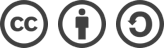## UVALive 6582 Magical GCD（数学+数据结构）解题报告

2017-02-20 1,889 次浏览

Also available: UVALive 6582, UVA 1642, Gym 100299C

i = 0
(30, 0)

i = 1
(30, 0)
(60, 1)

i = 2
(10, 0)
(20, 1)

i = 3
(10, 0)
(20, 1)

i = 4
(10, 0)
(20, 1)

## 参考代码

#include <cstdio>
#include <algorithm>
#include <stack>
#include <vector>
using namespace std;

long long GCD(long long a, long long b) {
return b ? GCD(b, a%b) : a;
}

int main(int argc, char const *argv[]) {
int t, n;
long long a;
scanf("%d", &t);
while(t--) {
scanf("%d", &n);
for(int i=0; i<n; ++i) {
scanf("%lld", &a[i]);
}
long long ans = a;
stack<pair<long long, int> > s; // 维护不同 GCD 的值及其最左端位置
s.push(make_pair(a, 0));
for(int i=1; i<n; ++i) {
vector<pair<long long, int> > v;
v.push_back(make_pair(a[i], i));
while(!s.empty()) {
v.push_back(make_pair(GCD(s.top().first, a[i]), s.top().second)); // 获得栈顶的 GCD 并计算新的 GCD，暂存到 v 中
s.pop();
}
for(int j=1; j<v.size(); ++j) { // 去除重复的 GCD 值
if(v[j].first == v[j-1].first)
v.erase(v.begin()+(j--)-1);
}
for(int j=v.size()-1; j>=0; --j) { // 将新的一组不同 GCD 值重新放回栈内并更新答案
ans = max(ans, v[j].first*(i-v[j].second+1));
s.push(v[j]);
}
}
printf("%lld\n", ans);
}

return 0;
}bLue 创作，采用 知识共享署名 3.0，可自由转载、引用，但需署名作者且注明文章出处。